Search

Partial-Fraction Decomposition:
General Techniques
(page 1 of 3)

Sections: General techniques, How to handle repeated and irreducible factors, Examples

Previously, you have added and simplified rational expressions, such as: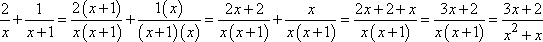Partial-fraction decomposition is the process of starting with the simplified answer and taking it back apart, of "decomposing" the final expression into its initial polynomial fractions.

To decompose a fraction, you first factor the denominator. Let's work backwards from the example above. The denominator is x2 + x, which factors as x(x + 1).

Then you write the fractions with one of the factors for each of the denominators. Of course, you don't know what the numerators are yet, so you assign variables (usually capital letters) for these unknown values: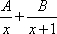Then you set this sum equal to the simplified result: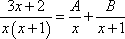Multiply through by the common denominator of x(x + 1) gets rid of all of the denominators: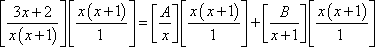Multiply things out, and group the x-terms and the constant terms:

3x + 2 = Ax + A1 + Bx
3x + 2 = (A + B)x + (A)1

(3)x + (2)1 = (A + B)x + (A)1

For the two sides to be equal, the coefficients of the two polynomials must be equal. So you "equate the coefficients" to get:

3 = A + B
2 = A

This creates a system of equations that you can solve:

A = 2
B = 1

Then the original fractions were (as we already know) the following: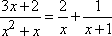There is another method for solving for the values of A and B. Since the equation "3x + 2 = A(x + 1) + B(x)" is supposed to be true for any value of x, we can pick useful values of x, plug-n-chug, and find the values for A and B. Looking at the equation "3x + 2 = A(x + 1) + B(x)", you can see that, if x = 0, then we quickly find that 2 = A:

3x + 2 = A(x + 1) + B(x)
3(0) + 2 = A(0 + 1) + B(0)
0 + 2 = A(1) + 0

2 = A

And if x = 1, then we easily get 3 + 2 = B, so B = 1.

I've never seen this second method in textbooks, but it can often save you a whole lot of time over the "equate the coefficients and solve the system of equations" method that they usually teach.

If the denominator of your fraction factors into unique linear factors, then the decomposition process is fairly straightforward, as shown in the previous example. But what if the factors aren't unique or aren't linear?

Top  |  1 | 2 | 3  |  Return to Index  Next >>

 Cite this article as: Stapel, Elizabeth. "Partial-Fraction Decomposition: General Techniques."     Purplemath. Available from https://www.purplemath.com/modules/partfrac.htm.     Accessed [Date] [Month] 2016

Study Skills Survey

Tutoring from Purplemath
Find a local math tutor